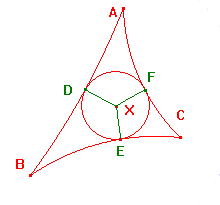# More theorems about hyperbolic triangles

 The Converse of Ceva's Theorem allows us to prove some familiar looking results in hyperbolic geometry Definition An h-median of a hyperbolic triangle is an h-segment joining a vertex to the h-midpoint of the opposite side. The Medians Theorem for Hyperbolic Triangles The h-medians of a hyperbolic triangle are concurrent. The figure on the right is a CabriJava illustration of the Medians Theorem. You can move the vertices A, B and C (within the boundary circle). The points P, Q and R are the h-midpoints of the sides of the h-triangle ABC.

 The Angle Bisectors Theorem for Hyperbolic Triangles The internal angle bisectors of a hyperbolic triangle are concurrent. As in euclidean geometry, the point of concurrence of the angle bisectors is the centre of the incircle - a circle touching all three sides. Indeed, the same proof suffices. In euclidean geometry, we then look at excircles - that is to say circles which touch all three sides of a triangle, but lie outside the triangle. These are best by looking at external bisectors of the angles. If we try this in the hyperbolic plane, we may find that the h-lines do not intersect, and no excircle exists. In these theorems, the h-segments all lie within the h-triangle, so that any two must meet. Then the Converse of Ceva's Theorem gives an unconditional result. In situations where two of the h-segments lie outside the h-triangle, we can have cases where the product of the h-ratios is 1, but the h-segments do not meet. A simple example is that of (hyperbolic) altitudes.Definition An h-altitude of a hyperbolic triangle is an h-segment through a vertex perpendicular to the opposite side. The Altitudes Theorem for Hyperbolic Triangles If any two h-altitudes of a hyperbolic triangle meet, then the h-altitudes are concurrent. The figure on the right is a CabriJava illustration of the Altitudes Theorem. You can move the vertices A, B and C (within the boundary circle). The points P, Q and R are the feet of the h-altitudes of the h-triangle ABC. By experimenting, you should be able to see that (1) there are cases where no two meet, and that (2) these occur only in certain cases where the h-triangle has an obtuse angle.

Many other euclidean theorems have hyperbolic analogues. For example, Stewart's Theorem,
and van Obel's Theorem.

In experiments with circles, we looked at the existence of a hyperbolic circle
through three points - the circumcircle of a triangle. We can now study this in detail.